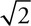# AP Physics 1 Question 107: Answer and Explanation

### Test Information

Question: 107

7.Four identical lead balls with large mass are connected by rigid but very light rods in the square configuration shown in the preceding figure. The balls are rotated about the three labeled axes. Which of the following correctly ranks the rotational inertia I of the balls about each axis?

• A. IB > IA = IC
• B. IA > IC = IB
• C. IC > IA > IB
• D. IC > IA = IB

D-The rotational inertia of a point mass is MR2, where R is the distance from the mass to the axis of rotation. Pretend the side of the square is of length 2 m, and that each mass is 1 kg. For axis A, each mass has rotational inertia (1 kg) (1 m)2 = 1 kg·m2. With four masses total, that's 4 kg·m2. For axis B, each mass ism from the axis (the diagonal of the square is 2m, each mass is half a diagonal from the axis). Each mass has (1 kg)(m)2 = 2 kg·m2. Two masses make a total of 4 kg·m2. And for axis C, the masses are each 2 m from the axis, so they each have (1 kg) × (2 m)2 = 4 kg·m2. With two masses, that's a total of 8 kg·m2. So this would be ranked axis C, followed by equal axes A and B.